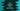# Dart HashSet fold and reduce methods explanation with examples## How to use the fold and reduce methods of Dart HashSet:

Both fold and reduce methods are used to get a single value from a HashSet. Both of these methods are different. In this post, we will learn how to use these methods with example programs.

### HashMap.reduce method:

The reduce method reduces one HashSet to a single value. It takes one function as its parameter to combine the results. It uses the function to iteratively combine the values to get the final result.

The syntax of the reduce method is:

``reduce(E combine(E v, E e)) → E``

Here, the combine function is the function that the reduce function uses to combine the values. The first parameter, v is the current calculated value and the second parameter e is the current iterating value.

For example:

``````import 'dart:collection';

void main() {
final hashSet = HashSet.of([1, 2, 3, 4, 5, 6, 7, 8, 9]);

final reducedValue = hashSet.reduce((v, e) => v + e);

print('Original HashSet: \${hashSet}');
print('Reduced value: \${reducedValue}');
}``````

In this example, the reduced function returns the sum of both the current value and the current calculated value. Finally, the reducedValue variable will hold the sum of all the numbers of the HashSet.

If you run this program, it will print:

``````Original HashSet: {1, 2, 3, 4, 5, 6, 7, 8, 9}
Reduced value: 45``````

To understand how this program works, let me modify the above program:

``````import 'dart:collection';

int combine(int v, int e) {
print('v: \${v}, e: \${e}');
return v + e;
}

void main() {
final hashSet = HashSet.of([1, 2, 3, 4, 5, 6, 7, 8, 9]);

final reducedValue = hashSet.reduce(combine);

print('Original HashSet: \${hashSet}');
print('Reduced value: \${reducedValue}');
}``````

We added a new function combine and passed this function to the HashSet.of method. The values of v and e are printed in the function and the sum of these values is returned from the function.

If you run this program, it will print:

``````v: 1, e: 2
v: 3, e: 3
v: 6, e: 4
v: 10, e: 5
v: 15, e: 6
v: 21, e: 7
v: 28, e: 8
v: 36, e: 9
Original HashSet: {1, 2, 3, 4, 5, 6, 7, 8, 9}
Reduced value: 45``````
• The first element is passed as the first parameter and the second parameter is the second parameter.
• The first element is the last step calculated value and the second element is the current value.
• It starts the iteration from the second element.
• The final calculated value is returned from the function.

### HashMap.fold method:

The fold method is similar to the reduce method. But it takes one initial value and that value is used with the combine function. The syntax of this method is:

``fold<T>(T initial, T combine(T previous, E element)) → T``

Here,

• The initial parameter is the initial value.
• The combine function is used to combine the values. Here, the previous parameter is the previous calculate value and the element parameter is the current element.

For example,

``````import 'dart:collection';

void main() {
final hashSet = HashSet.of([1, 2, 3, 4, 5, 6, 7, 8, 9]);

final foldValue =
hashSet.fold(10, ((previousValue, element) => previousValue + element));

print('Original HashSet: \${hashSet}');
print('Fold value: \${foldValue}');
}``````

Here,

• The initial value is 10 and the combine function is returning the sum of the previous value and the current value.

If you run this program, it will print:

``````Original HashSet: {1, 2, 3, 4, 5, 6, 7, 8, 9}
Fold value: 55``````

The sum of the values of the HashSet is 45 and since the initial value is 10, the final fold value is 55.

Let’s use a different function to understand how it works:

``````import 'dart:collection';

int combine(int previous, int element) {
print("Previous: \${previous}, element: \${element}");
return previous + element;
}

void main() {
final hashSet = HashSet.of([1, 2, 3, 4, 5, 6, 7, 8, 9]);

final foldValue = hashSet.fold(10, combine);

print('Original HashSet: \${hashSet}');
print('Fold value: \${foldValue}');
}``````

We created a new function combine and that function is printing the previous and the element values. It returns the sum of these two values.

It will print:

``````Previous: 10, element: 1
Previous: 11, element: 2
Previous: 13, element: 3
Previous: 16, element: 4
Previous: 20, element: 5
Previous: 25, element: 6
Previous: 31, element: 7
Previous: 38, element: 8
Previous: 46, element: 9
Original HashSet: {1, 2, 3, 4, 5, 6, 7, 8, 9}
Fold value: 55``````
• The previous value is the initial value we passed to the combine function.
• On each step, it returns the calculated value as the previous parameter and the current value as the element.
• It iterates over all the numbers of the HashSet.